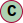# Class Orientation

Orientation quaternion with accuracy

#### Hierarchy-Diagram##### Legendclass

## Properties

_onChangeCallback: (() => void)

• (): void
• #### Returns void

accuracy: Accuracy<AngleUnit, number>
isQuaternion: true
timestamp: number
w: number
x: number
y: number
z: number

## Methods

• #### Parameters

• ##### callback: (() => void)
• (): void

• #### Returns Quaternion

• Copies values of q to this quaternion.

#### Returns boolean

• Sets this quaternion's x, y, z and w value from the provided array or array-like.

#### Parameters

• ##### array: number[] | ArrayLike<number>

the source array or array-like.

• ##### `Optional` offset: number

(optional) offset into the array. Default is 0.

#### Returns Orientation

• Sets x, y, z, w properties of this quaternion from the attribute.

#### Parameters

• ##### attribute: BufferAttribute | InterleavedBufferAttribute

the source attribute.

• ##### index: number

index in the attribute.

• #### Returns Quaternion

• Inverts this quaternion.

#### Returns Quaternion

• Computes length of this quaternion.

• #### Returns number

• Multiplies this quaternion by b.

• ### Deprecated

Use Vector#applyQuaternion vector.applyQuaternion( quaternion ) instead.

#### Returns any

• Normalizes this quaternion.

#### Returns Quaternion

• Sets values of this quaternion.

#### Returns Quaternion

• Sets this quaternion from rotation specified by Euler angles.

#### Returns Quaternion

• Returns an array [x, y, z, w], or copies x, y, z and w into the provided array.

### Returns

The created or provided array.

#### Parameters

• ##### `Optional` array: number[]

(optional) array to store the quaternion to. If this is not provided, a new array will be created.

• ##### `Optional` offset: number

(optional) optional offset into the array.

#### Returns number[]

• Copies x, y, z and w into the provided array-like.

### Returns

The provided array-like.

#### Parameters

• ##### array: ArrayLike<number>

array-like to store the quaternion to.

• ##### `Optional` offset: number

(optional) optional offset into the array.

#### Returns ArrayLike<number>

• Convert the quaternion to euler angles

### Returns

Converted euler

#### Parameters

• ##### `Optional` order: EulerOrder

Euler order

#### Returns Euler

• Convert axis angle rotation to quaternion

### Returns

Serializable quaternion

#### Parameters

• ##### axis: { angle?: number; unit?: AngleUnit; x: number; y: number; z: number; }

Axis-angle rotation

#### Returns InstanceType<T>

• Convert euler angles to quaternion

### Returns

Serializable quaternion

#### Parameters

• ##### euler: Vector3

Euler vector

#### Returns InstanceType<T>

• Convert rotation matrix to quaternion

### Returns

Serializable quaternion

#### Parameters

This type

• ##### matrix: Matrix4

Rotation matrix

#### Returns InstanceType<T>

• Convert a threejs quaternion to serializable quaternion

### Returns

Serializable quaternion

#### Parameters

• ##### threeQuaternion: Quaternion

ThreeJS created quaternion

• ### Deprecated

Use qm.slerpQuaternions( qa, qb, t ) instead..